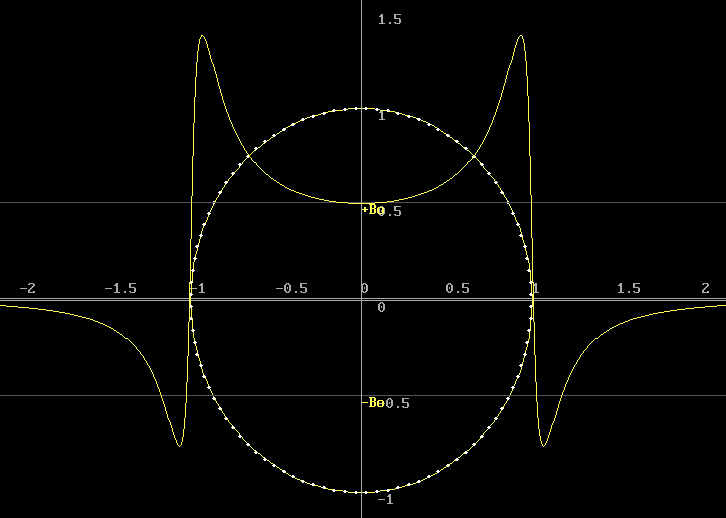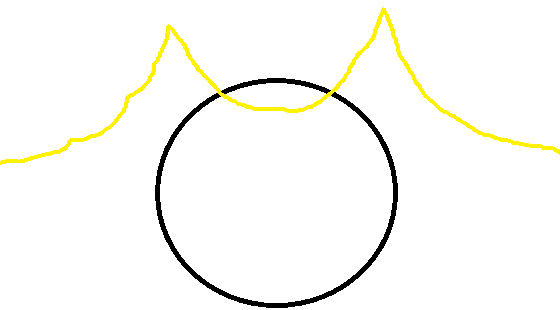# Biot-Savart non-textbook Equation -- B at point above a loop but off-axis

tim9000
I read everywhere about the formulas for calculating B at a point from a length of straight wire, or at a point from the centre of a closed loop.
But what about at a point over a closed loop that wasn't the centre? Is there a simple calculation for that?

Thanks

zoki85
tim9000
Thanks for the reply zoki, I only had a quick look, but didn't those examples still only deal with a point still being on the center (z) axis of the loop? (not like directly over the loop or closer to one side than the other)
I'll have a better look later, in which case if I'm wrong I'll remove this post.
Cheers

2021 Award
Attached is a plot of the field of a loop. This graph shows the magnetic field you would expect to measure as you walk across a wire loop resting on the ground surface. The loop is shown as the circle, viewed from above, modelled with many short segments as shown. The horizontal scale is in radii.
The plot drawn across the graph is the field that is seen travelling along a line that passes through the axis, parallel to, but slightly away from the plane of the loop. The plot is normalised so that +Bo is the axial field value.
The steepness of the transition while crossing the wire is determined by the offset from the plane of the coil. Let me know if you find an analytic solution.tim9000
I'm not sure I get it completely (cool graph by the way), I'm really sorry.
So there is a wire loop of current on the ground, I'm walking above it from -2 to +2, passing through the center of the axis of symmetry. I don't see how the yellow graph can be B? All I can picture is current traveling, say, anti-clockwise, then I would have through if the yellow graph was B, that as I approach it and am at -1 it peaks and all the B is coming from the LHS then as I am at the middle, the only component is from the center and there is some cancellation diagonally, then as I leave a gain at 1 it peaks with just RHS magnetic field. So why would it go negative as I first approach it, and leave why would it have a negative gradient? I would have thought it would taper up as I approach, peak, taper down (due to cancellation), taper up, peak, taper down.

Thanks heaps!

tim9000
So I thought it would look like this:2021 Award
Sorry, the graph is the vertical component of the field.

The vertical field inside the loop is opposite that outside the loop.

Over the wire the B field is horizontal so it has no vertical component.

tim9000
Ok that makes sense, but what if you wanted the total B strength, not just a component, over the path from left to right?
Would you sum the components, dot product, or cross product?
Cheers